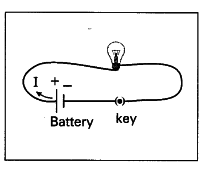# Conduct an activity to show that the resistance of a conductor is temperature dependent?

1. Take a bulb and measure the resistance of the bulb using a multimeter in open circuit. Note the value resistance.
2. Now connect the bulb in a circuit and switch on the circuit.
3. After few minutes the bulb gets heated.
4. Now measure the resistance of the bulb again with multimeter.
5. The value of resistance of the bulb in second instance is more than the resistance of the bulb in open circuit.
6. Here the increase in temperature of the filament in the bulb is responsible for increase in resistance of the bulb.
7. Thus the value of resistance of a conductor depends on the temperature.